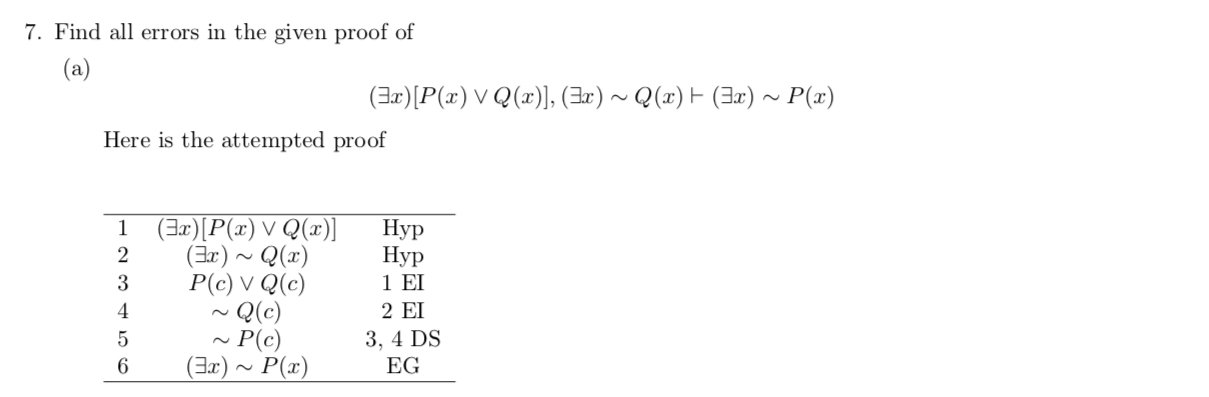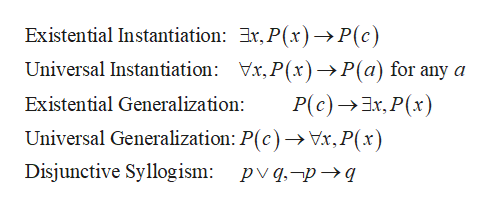# 7. Find all errors in the given proof of(a)(Ear)P(r) V Q(), (Ear') ~Q(x)(Ia)~ P(x)Here is the attempted proof(ErP(x) V Q(r)]2НурНур1 ΕΙ2 ΕΙ1(Er)Q(x)P(c) V Q(e)Q(c)P(c)(Ear)~P(x)343, 4 DSEG6

Question
104 viewshelp_outlineImage Transcriptionclose7. Find all errors in the given proof of (a) (Ear)P(r) V Q(), (Ear') ~Q(x) (Ia)~ P(x) Here is the attempted proof (ErP(x) V Q(r)] 2 Нур Нур 1 ΕΙ 2 ΕΙ 1 (Er)Q(x) P(c) V Q(e) Q(c) P(c) (Ear)~P(x) 3 4 3, 4 DS EG 6 fullscreen
check_circle

Step 1

Recall the follow...help_outlineImage TranscriptioncloseExistential Instantiation: Er, P(x)->P(c) Universal Instantiation: Vr,P(x)-»P(a) for any a P(c)x,P(x) Universal Generalization: P(c)-> Vx, P(x) Existential Generalization: _ Disjunctive Syllogism: pvq,-pq fullscreen

### Want to see the full answer?

See Solution

#### Want to see this answer and more?

Solutions are written by subject experts who are available 24/7. Questions are typically answered within 1 hour.*

See Solution
*Response times may vary by subject and question.
Tagged in

### Math